# How to Calculate Portfolio Risk and Return

In this article, we will learn how to compute the risk and return of a portfolio of assets. Let’s start with a two asset portfolio.

Portfolio Return

Let’s say the returns from the two assets in the portfolio are R1 and R2. Also, assume the weights of the two assets in the portfolio are w1 and w2. Note that the sum of the weights of the assets in the portfolio should be 1. The returns from the portfolio will simply be the weighted average of the returns from the two assets, as shown below:

RP = w1R1 + w2R2

Let’s take a simple example. You invested $60,000 in asset 1 that produced 20% returns and$40,000 in asset 2 that produced 12% returns. The weights of the two assets are 60% and 40% respectively.

The portfolio returns will be:

RP = 0.60*20% + 0.40*12% = 16.8%

Portfolio Risk

Let’s now look at how to calculate the risk of the portfolio. The risk of a portfolio is measured using the standard deviation of the portfolio. However, the standard deviation of the portfolio will not be simply the weighted average of the standard deviation of the two assets. We also need to consider the covariance/correlation between the assets. The covariance reflects the co-movement of the returns of the two assets. Unless the two assets are perfectly correlated, the covariance will have the impact of reduction in the overall risk of the portfolio.

The portfolio standard deviation can be calculated as follows: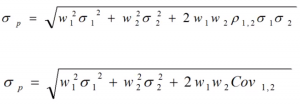In the above example, let’s say the standard deviation of the two assets are 10 and 16, and the correlation between the two assets is -1. The standard deviation of the portfolio will be calculated as follows:

σP = Sqrt(0.6^2*10^2 + 0.4^2*16^2 + 2*(-1)*0.6*0.4*10*16) = 0.4

Correlation and Covariance

As you can see, the standard deviation can be calculated using either covariance or correlation. The relationship between the two is depicted below: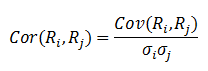In this example, we had taken a simple portfolio of two assets however as the number of assets in the portfolio increase, the complexity will increase as we will have to consider the covariance between each pair of the assets in the portfolio. For a three asset portfolio, the risk and return will be calculated as follows: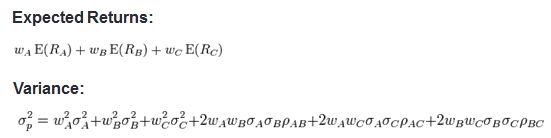The calculation can be simplified by representing the formula in the form of an equation. For a two asset portfolio the formula can be represented as: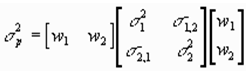Note that there are there matrices in the calculation. The first one is a one-dimensional matrix of the asset weights in the portfolio. The second matrix is called the variance covariance matrix with the variances of the assets as the diagonal elements and the covariance as the other elements. The third matrix is the transpose of the first matrix. Multiplying the three matrices gives us the variance of the portfolio. In Microsoft Excel, you can use the MMULT function to perform matrix multiplication.

This form of variance calculation can easily be expanded to as many assets as we want.

Let’s take the case of a three asset portfolio with asset weights as 0.3, 0.4, and 0.3. The variance covariance matrix is given below: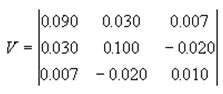The variance of the portfolio can be calculated as follows: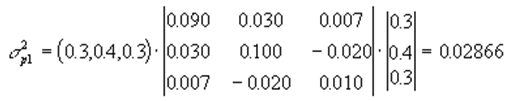Get our Data Science for Finance Bundle for just $29$51.